Algebra Fundamentals

While most approaches to algebra emphasize its mechanical rule-following nature, we will be working to develop an intuition for the principles that make algebra work.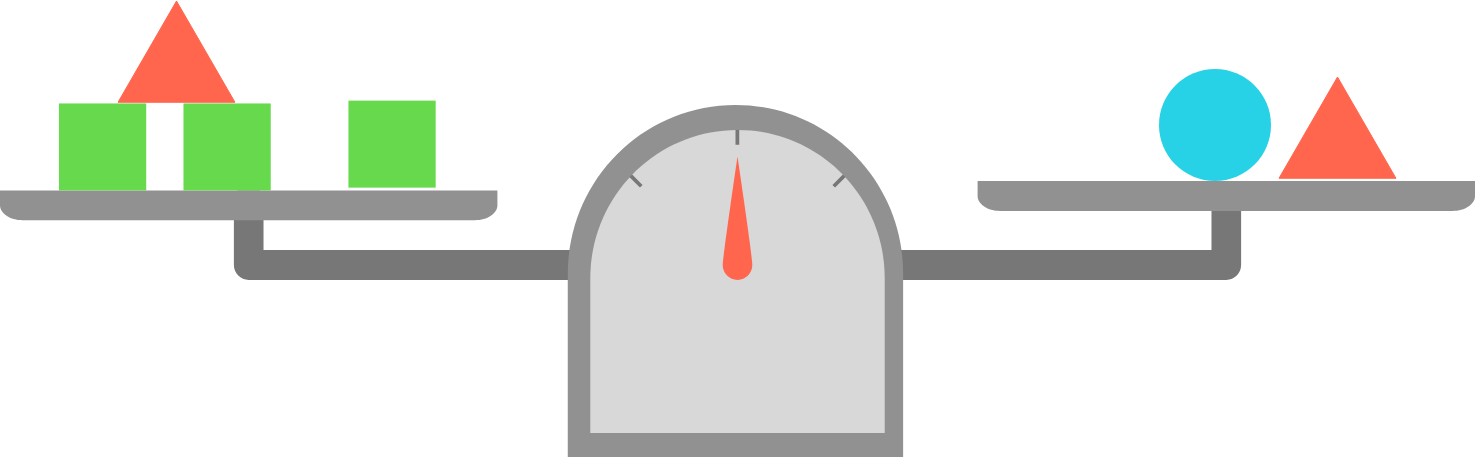In the upcoming problems, try to think about the problems as a physical system that you can manipulate. For example, in the image above, can you imagine what would happen to the scale if you removed only the blue circle? What if you removed both of the red triangles?

Developing this kind of intuition will help you become a stronger problem solver!

Scale and Lever Logic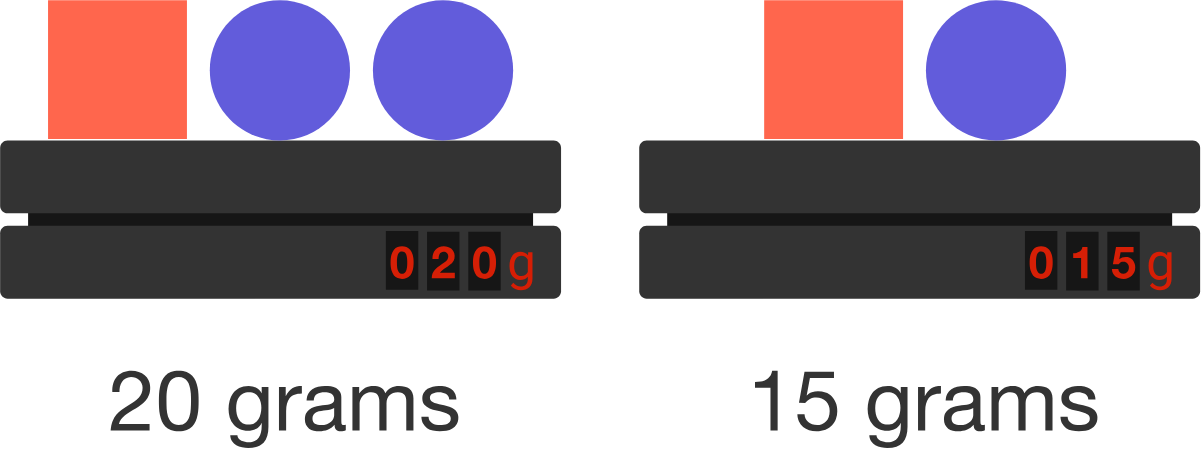What is the mass of a single red square?

Scale and Lever Logic

While this problem appears different, the same intuition you can use to solve balance scale problems can also be used here.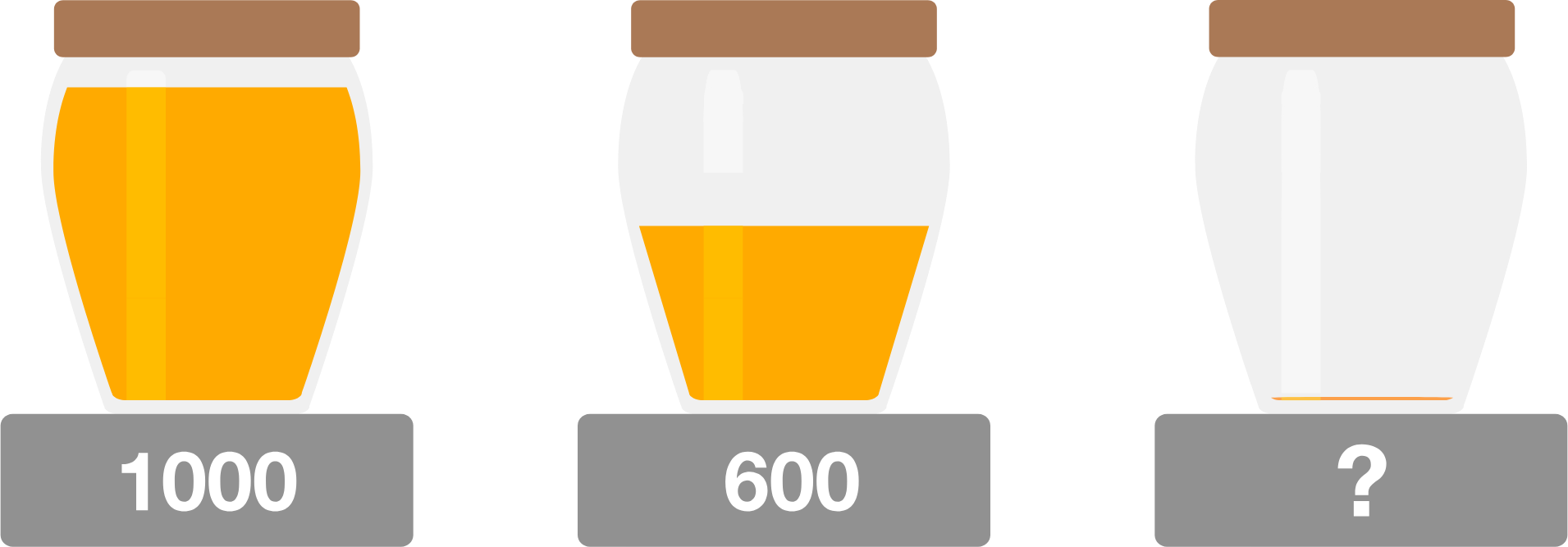• The mass of a full jar of honey is 1000 grams.
• With half of the honey, the total mass is 600 grams.

What is the mass of the empty jar in grams?

Scale and Lever Logic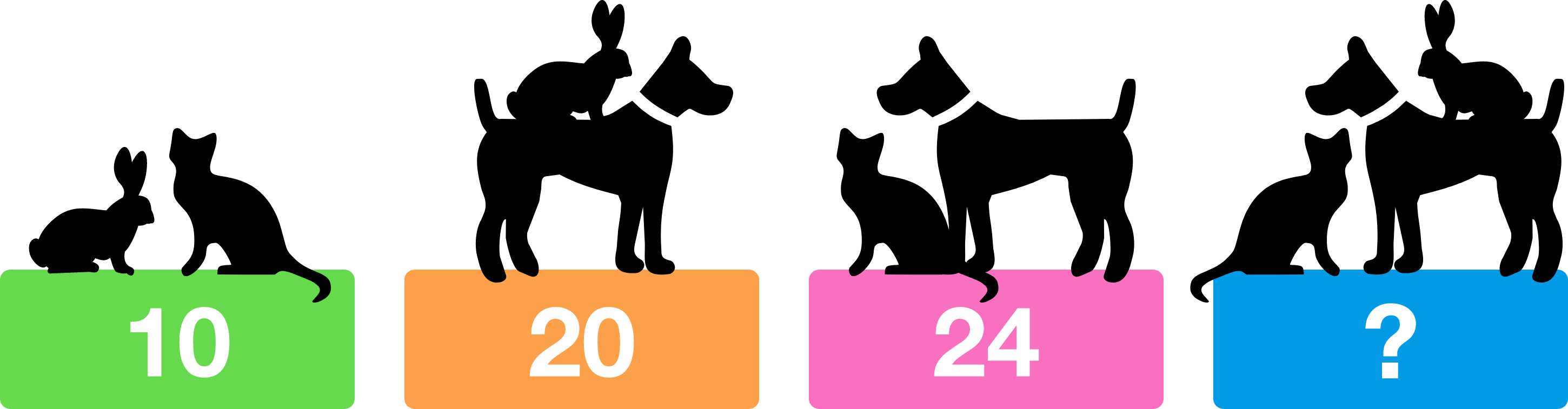What weight will the fourth scale display?

Think carefully! There's a quick and clever shortcut for solving this.

Scale and Lever Logic

At the end of this course, you'll have a thorough toolbox of algebraic techniques that feel natural to use and empower you to master complex problems and puzzles!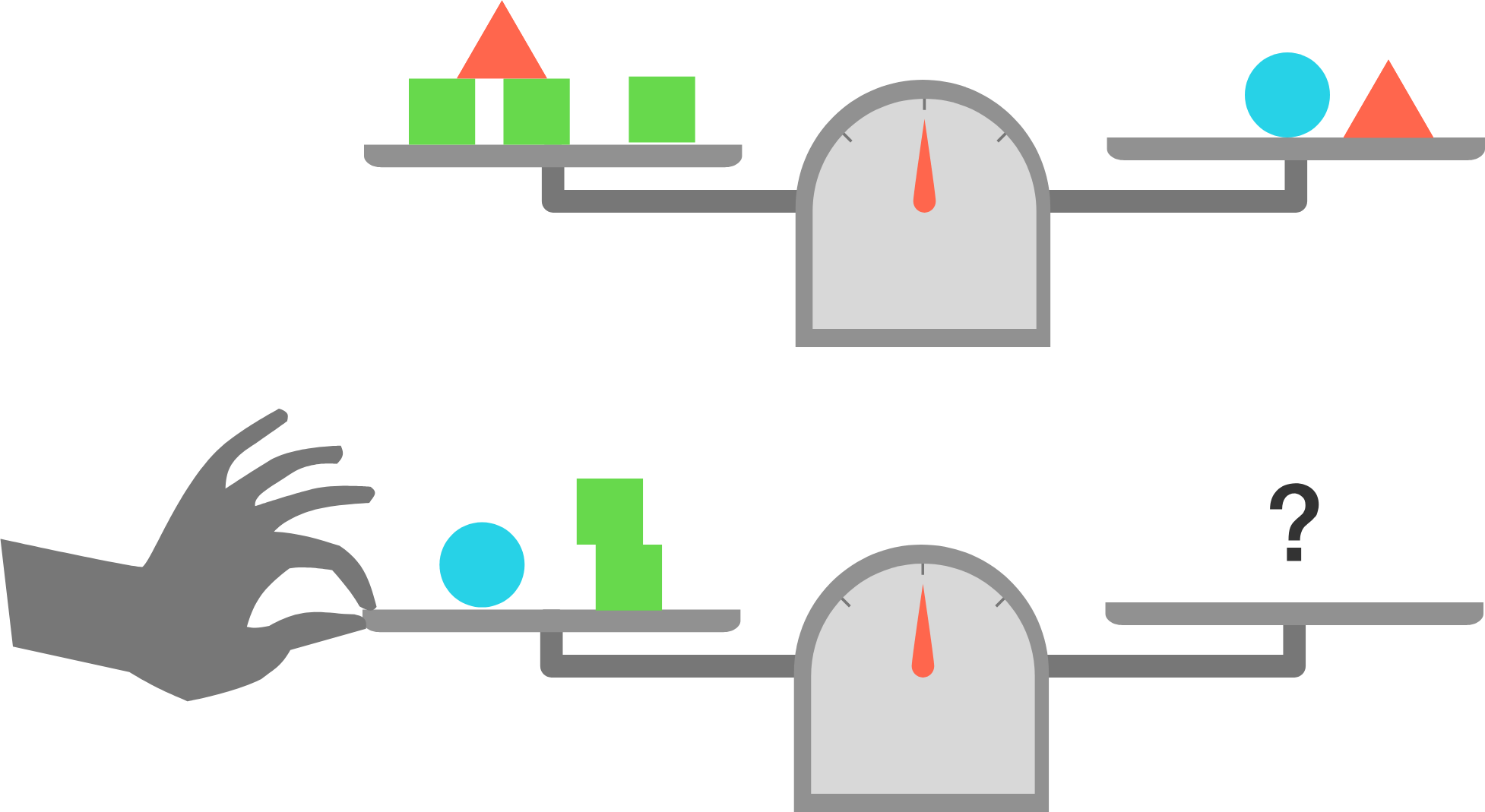How many green squares should be placed on the right side of the bottom scale in order to make it balance when released?

Scale and Lever Logic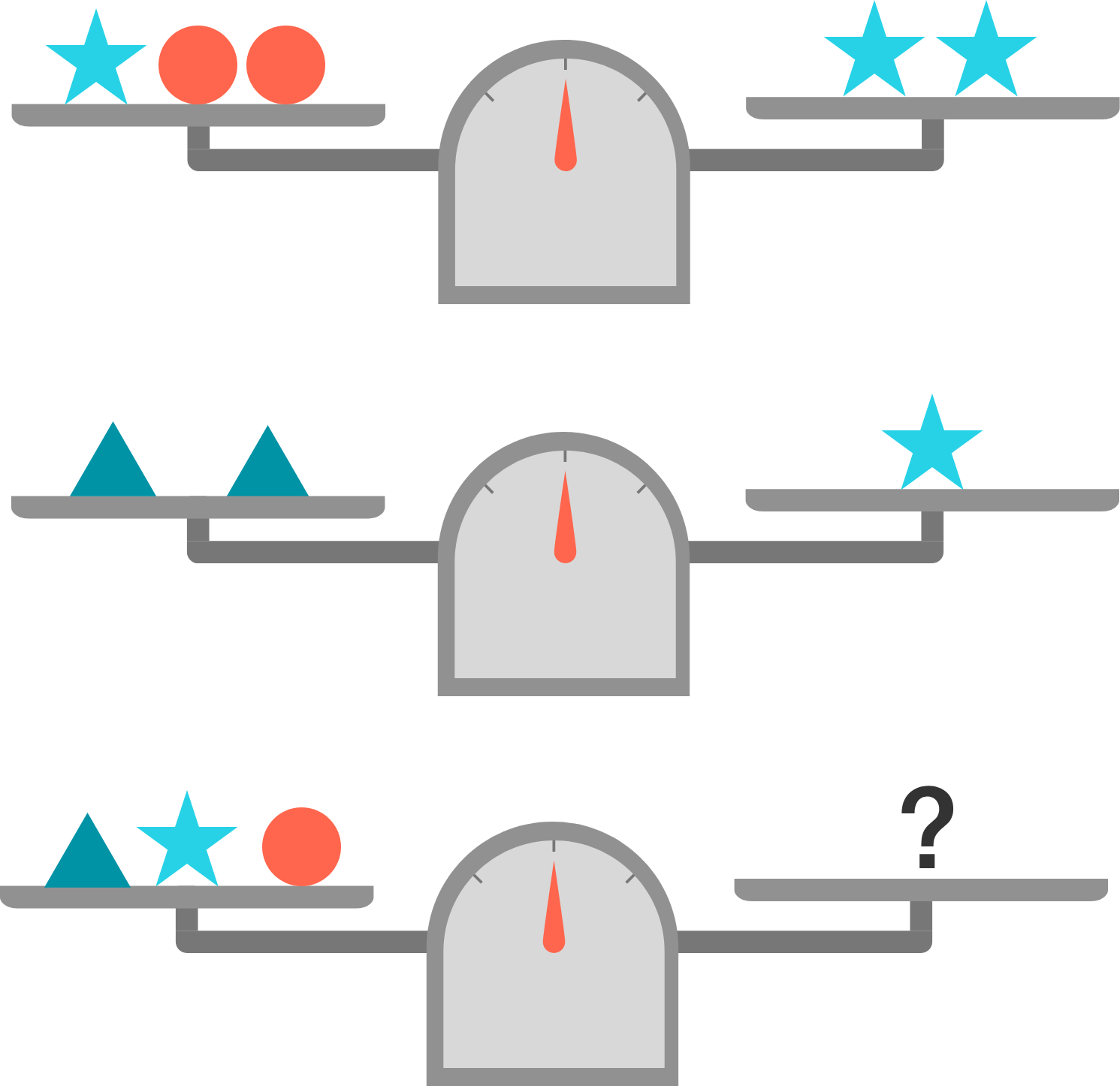How many red balls need to be added to the right hand side of the bottom scale to put it in balance?

×